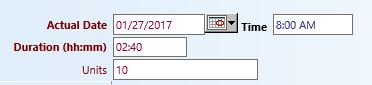## How To: Calculate Units Based on Duration

Many programs bill for units that are calculated based on duration. For example, programs may bill where 1 unit = 15 minutes of service time. The myEvolv Finance module handles those calculations to generate an accurate claim based on the duration entered in the service documentation but there may be times when you would like the calculated units to display on the form itself. There are no system form fields that do this for you but you can calculate the value with JavaScript and put it into a custom string field for display on the form.## Duration Fields

The first step is to obtain the value in the duration field and we want to do this every time the duration changes on the form so in the On Change code for the `duration` field, we will use the following code:

`var dur = getFormElement('duration');`

Duration fields have masked inputs. When you put in ’30’, the myEvolv converts that to `00:30`. When you put in ’90’, myEvolv converts it to `01:30`. Therefore when you go to retrieve the value in the field, you are given an HH:MM string. The first task in calculating units is to convert this value back to an integer representing the number of minutes.

## Split the String

We know the mask for the time is HH:MM so all we need to do is break the string into hours and minutes. We can do this using JavaScript’s split method:

`var a = dur.split(':');`

Here we have created a new variable that is an array containing hours and minutes. If the initial input was ’02:45′, `a` is now equal to an array that looks like this:

`('2', '45')`

## Calculate Minutes

Now we have to perform a little math to get the number of minutes.

`var mins = (+a * 60 + (+a));`

Here, we take the hours in `a` and multiply that by 60 to get the number of minutes and then add it to the minutes in `a`. The ‘+’ signs tell JavaScript to cast the values in the array as numerical values so that math can be performed on them. Remember that the value we got from the `duration` field was a string.

## Calculate Units

For the sake of this example, we will use 15 minutes = 1 unit. We simply need to divide `mins` by the number of minutes that makes 1 unit to get the number of units. However, there is one tricky bit. Often these billing scenarios do not allow for the billing of partial units. That means if the duration is ’00:35′, we want to calculate 2 units, not 2.333333333 units. So in addition to the division, we will also use JavaScript’s Math.floor method so that partial units are rounded down to the nearest whole.

`var units = Math.floor(mins / 15);`

If you need to calculate a different unit formula, you can do that by changing the number of minutes. For 1 hour = 1 unit, you just make the ’15’ into a ’60’.

## Set the Units Value

Finally, we just need to put the calculated units into our units field. I am using a custom string type field.

`setFormElement('udf_calculated_units', units);`

With this code in place for the On Change event on the `duration` field, the correct number of units should calculate into the `udf_calculated_units` field whenever the duration value changes.

### Full Code Block

`var dur = getFormElement('duration');`
`var a = dur.split(':');`
`var mins = (+a * 60 + (+a));`
`var units = Math.floor(mins / 15);`
`setFormElement('udf_calculated_units', units);`

## We are using cookies on our website

Please confirm, if you accept our tracking cookies. You can also decline the tracking, so you can continue to visit our website without any data sent to third party services.